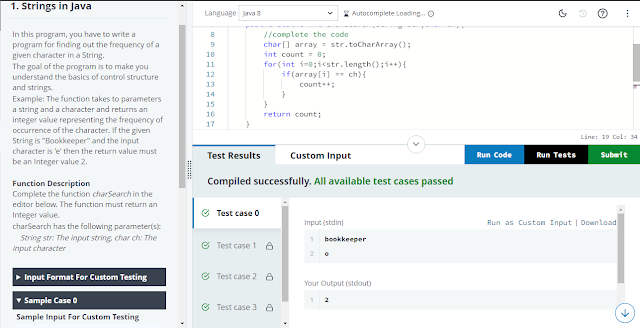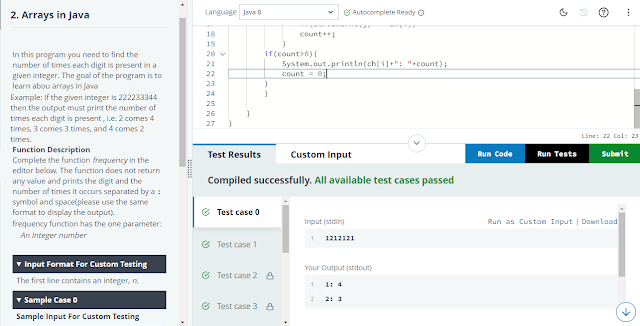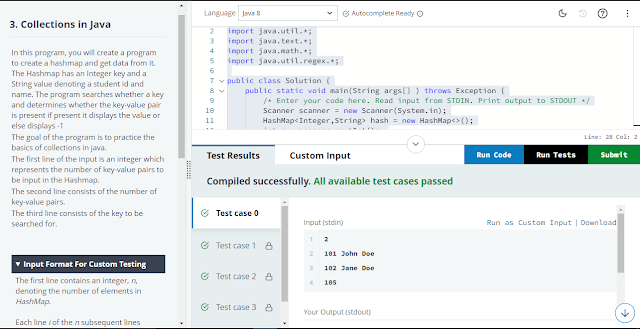# Mini Project Java Hands-On Solution | TCS Fresco Play

5

###Disclaimer: The primary purpose of providing this solution is to assist and support anyone who are unable to complete these courses due to a technical issue or a lack of expertise. This website's information or data are solely for the purpose of knowledge and education.Make an effort to understand these solutions and apply them to your Hands-On difficulties. (It is not advisable that copy and paste these solutions)

The Course Id: -  63716

### 1. Strings in Javaimport java.io.IOException;

public class Solution{

public static int charSearch(String str,char ch){
//complete the code
char[] array = str.toCharArray();
int count = 0;
for(int i=0;i<str.length();i++){
if(array[i] == ch){
count++;
}
}
return count;
}

public static void main(String[] args) throws IOException {

System.out.println(charSearch(str,ch));

}
}

### 2. Arrays in Javaimport java.io.*;
import java.util.*;
import java.text.*;
import java.math.*;
import java.util.regex.*;

public class Solution {
public static void main(String args[] ) throws Exception {
/* Enter your code here. Read input from STDIN. Print output to STDOUT */
Scanner scanner = new Scanner(System.in);
char[] ch = {'0','1','2','3','4','5','6','7','8','9'};
int a = scanner.nextInt();
String str = Integer.toString(a);
int count = 0;
for(int i=0;i<ch.length;i++){
for(int j=0;j<str.length();j++){
if(str.charAt(j) == ch[i])
count++;
}
if(count>0){
System.out.println(ch[i]+": "+count);
count = 0;
}
}

}
}

### 3. Collections in Java:-import java.io.*;
import java.util.*;
import java.text.*;
import java.math.*;
import java.util.regex.*;

public class Solution {
public static void main(String args[] ) throws Exception {
/* Enter your code here. Read input from STDIN. Print output to STDOUT */
Scanner scanner = new Scanner(System.in);
HashMap<Integer,String> hash = new HashMap<>();
int n = scanner.nextInt();
scanner.nextLine();
while(n>0){
String str =scanner.nextLine();
String s[] = str.split(" ");
hash.put(Integer.parseInt(s), s);
n--;
}
int k = scanner.nextInt();
if(hash.containsKey(k)){
System.out.println(hash.get(k));
}
else{
System.out.println("-1");
}
}
}

If you have any queries, please feel free to ask on the comment section.

If you want MCQs and Hands-On solutions for any courses, Please feel free to ask on the comment section too.

1.Mini-project - Bot Intent Classification

2.MongoDB cartula inceptives handson

3.1.We will upload ASAP. Thanks!

4.import java.io.IOException;

public class Solution{

public static int charSearch(String str,char ch){
//complete the code
char[] array = str.toCharArray();
int count = 0;
for(int i=0;i<str.length();i++){
if(array[i] == ch){
count++;
}